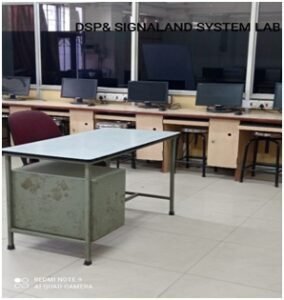### ECE Department

Introduction

Digital Signal Processing (DSP) Lab deals with generating practical exposure for students on techniques of processing discrete signals on the basis of their frequency components, the analysis and design of their filtration techniques and the method of their structural representation. Here, the aim is to extend the student’s knowledge of discrete signals and systems to an improved understanding of practical data processing while introducing them to classical signal processing techniques which are still widely relevant in practical day to day life.

Objective of the Laboratory

The objective of the laboratory is to enable the students-

• To learn via simulation the mathematical approach of simulating all of the concepts studied in the subject theory.
• To introduce the students to MATLAB as a DSP algorithm implementation tool and demonstrate the power of the system with the inbuilt function usage and hands-on algorithm development
• To critically analyze the limitations of the simulation platforms and understand the gaps between the theory, the simulation and the practical

Courses to cater

• ETEC 356 Digital Signal Processing Lab

Facilities

• Software – MATLAB

Current Research Projects

• None

People

• Faculty Coordinator: Dr. Rubeena Vohra
• Other Faculty Users: Mr. Abhishek Gagneja, Ms. Suman Yadav
• Lab Technician: Ms. Deepa Bhatia